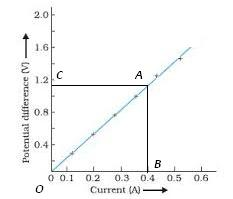you are here->home->Physics->Class 10->Ohm's Law
Ohm's Law

# Our Objective

Our objective is to determine the resistance per centimeter of a given wire by plotting a graph of potential difference versus electric current.

# The Theory

According to the Ohm's law, "The electric current flowing through a conductor is directly proportional to the potential difference across its ends provided the physical conditions (temperature, dimensions, pressure) of the conductor remains the same."

If I is the current flowing through a conductor and V is the potential difference across its ends, then according to Ohm's Law,where, R is the constant of proportionality. It is known as resistance of the conductor, orR depends upon the material, temperature and dimensions of the conductor.

In S.I. units, the potential difference 'V' is measured in volt and the current 'I' in ampere, the resistance 'R' is measured in ohm.To establish the current-voltage relationship, it is to be shown that the ratio V / I remains constant for a given resistance and therefore a graph between the potential difference(V) and the current (I) must be a straight line.

The value of unknown resistance can be calculated using the equation ,Here R is a constant.

To find the length of the resistance wire, l. ThenThis gives the resistance per unit cm.

## Learning Outcomes

• Students learn Ohm’s law.
• Students know the relation between voltage, current and resistance.

Cite this Simulator: